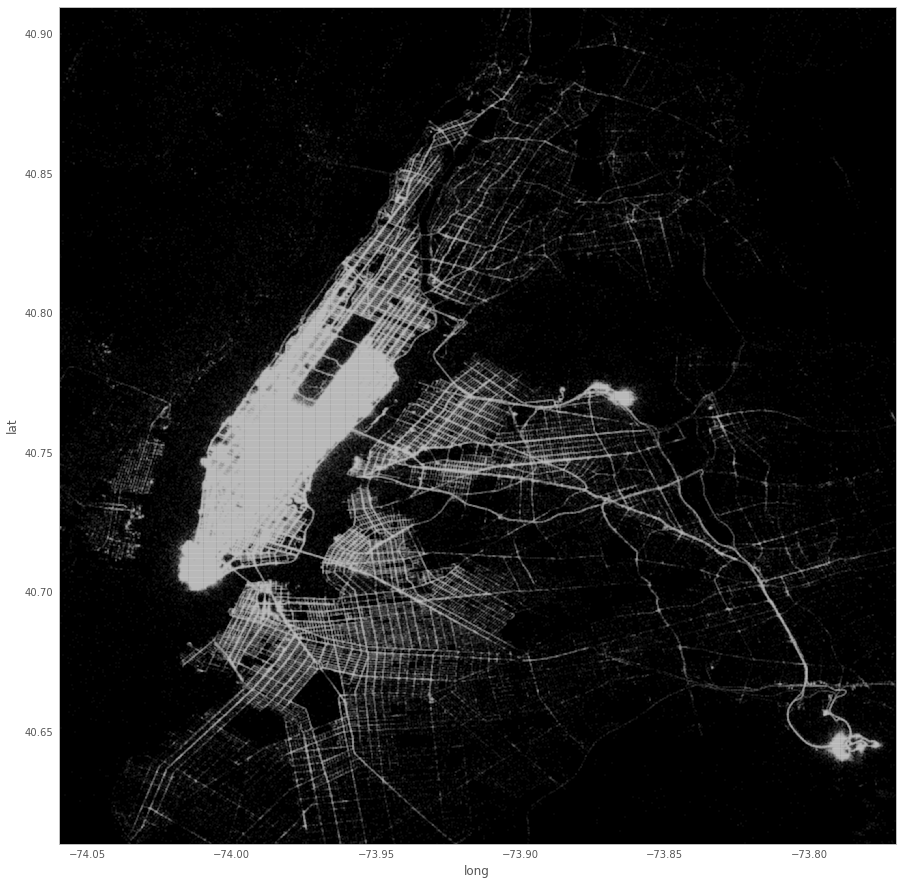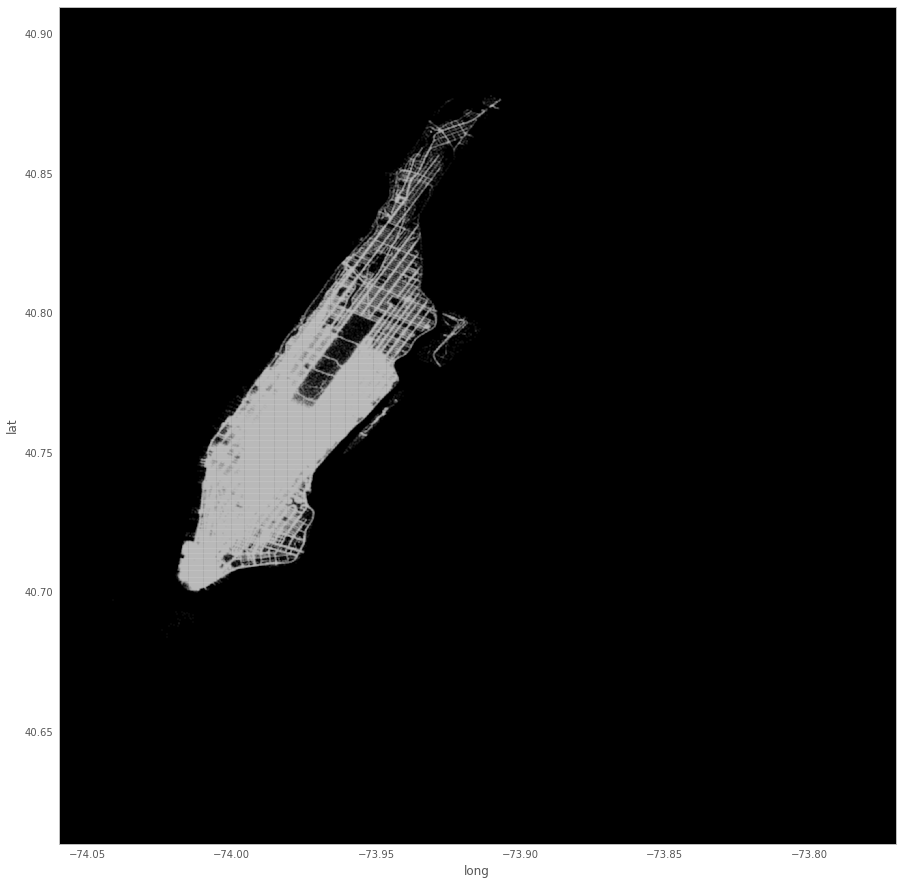In this post I'll take a try at using NYC's publicly available taxi data, first by accessing it via Google's BigQuery and plotting the results as seen in this post. Then I will label whether each lon/lat combination is within Manhattan and plot only the pickups that are within Manhattan.

## Fetching and plotting the data¶

We will follow the the instructions found here. The code is either identically or heavily inspired from that post.

In :
import pandas as pd

SELECT ROUND(pickup_latitude, 4) as lat, ROUND(pickup_longitude, 4) as long, COUNT(*) as num_pickups
FROM [nyc-tlc:yellow.trips]
WHERE (pickup_latitude BETWEEN 40.61 AND 40.91)
AND (pickup_longitude BETWEEN -74.06 AND -73.77 )
AND (YEAR(pickup_datetime) == 2015)
GROUP BY lat, long
""", project_id='taxi-data-ramon')

Waiting for job to complete...

In :
import matplotlib
import matplotlib.pyplot as plt
#Inline Plotting for Ipython Notebook
%matplotlib inline

import warnings
warnings.filterwarnings('ignore')

pd.options.display.mpl_style = 'default' #Better Styling
new_style = {'grid': False} #Remove grid
matplotlib.rc('axes', **new_style)
from matplotlib import rcParams
rcParams['figure.figsize'] = (15, 15) #Size of figure
rcParams['figure.dpi'] = 125

P=df.plot(kind='scatter', x='long', y='lat',color='white',xlim=(-74.06,-73.77),ylim=(40.61, 40.91),s=.02,alpha=.6)

P.set_axis_bgcolor('black') #Background Color## Filtering pickups to Manhattan only¶

We are interested only in trips that were originated in Manhattan. For this we will make use of GeoPandas, and we will need a map of Mahattan. Let's fetch the map of Manhattan (from here) and plot it with Folium.

In :
import geopandas as gpd

#help functions
assets = '/'.join(os.getcwd().split('/')[:-1] + ['assets'])
path = (assets + '/{}').format


In :
import folium
import os

#let's center on the WTC
manhattan = folium.Map(location=[40.7118, -74.0105], zoom_start=11, tiles='Stamen Toner')

manhattan.geo_json(geo_str=manh_df.to_json().replace("'", r"\'"),
line_color='#3186cc',
fill_color='#3186cc', line_weight=2,
fill_opacity=0.3, reset=True)

In :
manhattan

Out:
In :
from shapely.geometry import Point

crs = None
geometry = [Point(xy) for xy in zip(df.long, df.lat)]
geo_df = gpd.GeoDataFrame(df, crs=crs, geometry=geometry)

In :
geo_df.head()

Out:
lat long num_pickups geometry
0 40.7110 -73.7924 1 POINT (-73.7924 40.711)
1 40.7797 -73.9265 1 POINT (-73.9265 40.7797)
2 40.7305 -73.8415 1 POINT (-73.8415 40.7305)
3 40.7556 -73.9462 1 POINT (-73.9462 40.7556)
4 40.8100 -73.8747 1 POINT (-73.8747 40.81)
In :
geo_df['in_manhattan'] = geo_df.intersects(manh_df['geometry'].unary_union)

In :
manhattan_points = geo_df[geo_df['in_manhattan']]


We've now filtered the points to include only those which are contained within Manhattan. We can redo the first plot.

In :
manhattan_points_df = pd.DataFrame(manhattan_points)
P = manhattan_points_df.plot(kind='scatter', x='long', y='lat',color='white',xlim=(-74.06,-73.77),ylim=(40.61, 40.91),s=.02,alpha=.6)

P.set_axis_bgcolor('black') #Background ColorNice. Next time I would like to try using "within" rather than "intersects" and perhaps plot a nice heatmap on an interactive map.